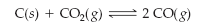×
Get Full Access to Introductory Chemistry - 5 Edition - Chapter 15 - Problem 71p
Get Full Access to Introductory Chemistry - 5 Edition - Chapter 15 - Problem 71p

×

# This reaction is endothermic. Predict the effect (shift right, shift left, or no effect)ISBN: 9780321910295 34

## Solution for problem 71P Chapter 15

Introductory Chemistry | 5th Edition

• Textbook Solutions
• 2901 Step-by-step solutions solved by professors and subject experts
• Get 24/7 help from StudySoup virtual teaching assistantsIntroductory Chemistry | 5th Edition

4 5 1 426 Reviews
24
5
Problem 71P

This reaction is endothermic.Predict the effect (shift right, shift left, or no effect) of these changes.

(a) increasing the reaction temperature

(b) decreasing the reaction temperature

Step-by-Step Solution:
Step 1 of 3

Solution: Here, we are going to predict the effect of the changes in the given reaction.

Step1:

Le Chatelier’s principle states that, a change in any of the factors that determine the equilibrium conditions of a system will cause the system to change in such a manner so as to reduce or to counteract the effect of the change.

For an exothermic reaction, increase in temperature decreases the value of the equilibrium constant and for an endothermic reaction, the equilibrium constant increases as the temperature increases.

Step2:

Since, the given reaction is endothermic, therefore,

1. Increasing the reaction temperature will shift the equilibrium to the right.
2. Decreasing the reaction temperature will shift the equilibrium to the left.

----------------------------

Step 2 of 3

Step 3 of 3

##### ISBN: 9780321910295

Unlock Textbook Solution